# Ryser's conjecture

 Importance: High ✭✭✭
 Author(s): Ryser, Herbert J.
 Subject: Graph Theory » Hypergraphs
 Keywords: hypergraph matching packing
 Posted by: mdevos on: March 19th, 2007
Conjecture   Letbe an-uniform-partite hypergraph. Ifis the maximum number of pairwise disjoint edges in, andis the size of the smallest set of vertices which meets every edge, then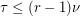.

Definitions: A (vertex) cover is a set of vertices which meets (has nonempty intersection with) every edge, and we letdenote the size of the smallest vertex cover of. A matching is a collection of pairwise disjoint edges, and we letdenote the size of the largest matching in. When the hypergraph is clear from context, we just writeor.

It is immediate that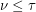, since every cover must contain at least one point from each edge in any matching. For-uniform hypergraphs,, since the union of the edges from any maximal matching is a set of at mostvertices that which meets every edge. Ryser's conjecture is that this second bound can be improved ifis-uniform and-partite (the vertices may be partitioned intosetsso that every edge contains exactly one element of each).

In the special case whenour trivial inequality yieldsand the conjecture implies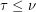, so we should have. In fact this is true, it is König's theorem on bipartite graphs [K]. Indeed, Ryser's conjecture is probably easiest to view as a high dimensional generalization of this early result of König. Recently, Aharoni [A] has applied the "Hall's theorem for hypergraphs" result of Aharoni and Haxell [AH] to prove this conjecture for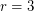. However the caseis still wide open.

Some other interesting work on this problem concerns fractional covers and fractional matchings. A fractional cover of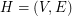is a weighting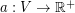so that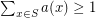for every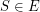, and the weight of this cover is. The fractional cover number, denotedis the infimum of the set of weights of covers. Similarly, a fractional matching is an edge-weighting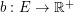so thatfor every, and the weight of this matching is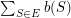. The fractional matching number, denoted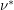is the supremum of the set of weights of fractional matchings. Fractional covers and matchings are the usual fractional relaxations, and by LP-duality, they satisfyfor every hypergraph. For-regular-partite hypergraphs, Füredi [F] has proved that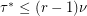and Lovasz [L] has shown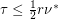.

## Bibliography

[A] R. Aharoni, Ryser's conjecture for tripartite 3-graphs. Combinatorica 21 (2001), no. 1, 1--4. MathSciNet

[AH] R. Aharoni and P. Haxell, Hall's theorem for hypergraphs. J. Graph Theory 35 (2000), no. 2, 83--88. MathSciNet

[F] Z. Füredi, Maximum degree and fractional matchings in uniform hypergraphs, Combinatorica 1 (1981), 155--162. MathSciNet

[K] D. König, Theorie der endlichen und unendlichen Graphen, Leipzig, 1936.

[L] L. Lovász, On minimax theorems of combinatorics, Ph.D thesis, Matemathikai Lapok 26 (1975), 209--264 (in Hungarian). MathSciNet

* indicates original appearance(s) of problem.